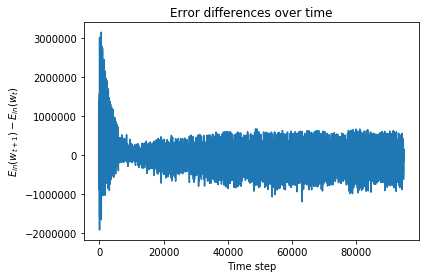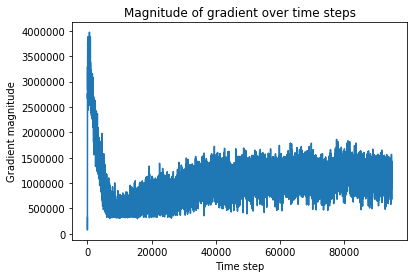CoCalc Public FilesML_Spr_20 / Homeworks / HW3_GRDDES / HW3.html
Author: Hunter Johnson
Views : 96
Compute Environment: Ubuntu 18.04 (Deprecated)
HW3

### Homework 3¶

In this homework we will implement a basic version of gradient descent.

Below, look for unfinished functions that contain a line like

## You complete me

Where those occur, fill in the missing code.

When your code is correct the output should be similar to the output provided (see the HTML version of this document for backup output images).

As in all our exercises, please be sure your function works for input that has any dimensionality (changing $d$ and $N$ should not break the code).

Reminder: It's cheating to google the solution and use the code you find. Don't pollute your brain by googling. Just try to solve the problem.

In :
import numpy as np
import matplotlib.pyplot as plt
import pandas as pd

### Here is the function you must complete
### Below you can see some tests which you can use to determine if you've succeeded.
### In other words you don't need to implement fancy stopping conditions.

optional: eta (default 0.1) max_iter (default 1000)
"""
history=[]  ## Each time a new w is considered, add it to this list
history.append(w) ## Just like this
for i in range(max_iter):
### You complete me
history.append(w)
return w,np.array(history)

In :
### First we'll try the function on a made up regression problem.

from sklearn import datasets

X_no_bias,y = datasets.make_regression(n_features=1,noise=10,random_state=100)

plt.scatter(X_no_bias,y,alpha=0.6)
plt.show()

Out: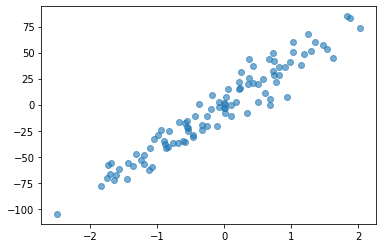In :
## Add a bias column
X = np.c_[np.ones(X_no_bias.shape),X_no_bias]
X[:5], X.shape,y.shape

Out:
(array([[ 1.        , -0.37690335],
[ 1.        , -1.1994512 ],
[ 1.        , -1.74976547],
[ 1.        ,  0.98132079],
[ 1.        , -1.70595201]]), (100, 2), (100,))
In :
## Get a train test split of the data

from sklearn.model_selection import train_test_split
X_train,X_test,y_train,y_test = train_test_split(X,y)


### Linear regression with the normal equations¶

As we've said in class, linear regression with the normal equations is a one-liner.

Please write that line in the cell below.

You want

$\bar{w} = X_{train}^\dagger y_{train}$

where $X_{train}^\dagger$ is the pseudo-inverse of $X_{train}$.

In :
w = ### You complete me

y_train_hat = X_train.dot(w)
plt.scatter(X_train[:,1],y_train)
plt.plot(X_train[:,1],y_train_hat,c='r')
plt.title("The regression line learned by the normal eqns")
plt.show()

Out: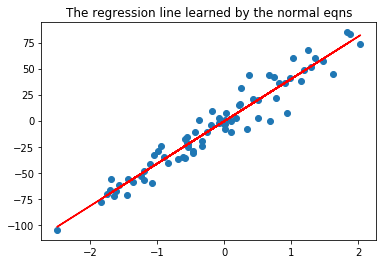### Assess performance¶

Below we use $R^2$ to assess the performance of the model.

Please help us out by implementing the R2 function.

You can remind yourself of the definition here:

https://en.wikipedia.org/wiki/Coefficient_of_determination#Definitions

In :
def R2(y,yhat):
"""parameters: y, yhat"""
## You complete me

y_test_hat = X_test.dot(w)

print("w = {}".format(w))
print("R2 = {}".format(R2(y_test,y_test_hat)))

w = [-0.67945656 40.67325542]
R2 = 0.8905318861468269


Now let's try to find the solution to this same problem using gradient descent.

You'll need the gradient of the mean squared error function which is on page 85 of the textbook.

$\nabla E_{in}(\bar{w}) = \frac{2}{N}(X^TX\bar{w}-X^Ty).$

Remember that the computer is not psychic and will not understand "N" which is the number of rows in $X$.

In :
def mse_gradient(w,X,y):
## You complete me
## This formula is given above

In :
w_init = np.random.randn(2)/10

y_test_hat = X_test.dot(wgd)

print("w (normal eqn) = {}".format(w))
print("R2 = {}".format(R2(y_test,y_test_hat)))

w (normal eqn) = [-0.67945656 40.67325542]
w (gradient descent) = [-0.67945656 40.67325542]
R2 = 0.8905318861468269

In :
## First 7 w's visited in grad descent
path[:7]

Out:
array([[-0.23387852, -0.06264982],
[-1.49889639,  8.47135849],
[-2.2645639 , 15.18370436],
[-2.68333609, 20.46985804],
[-2.86576119, 24.63816441],
[-2.89137693, 27.92927751],
[-2.81686666, 30.53121539]])
In :
## Last 5 w's visited

path[-5:]

Out:
array([[-0.67945656, 40.67325542],
[-0.67945656, 40.67325542],
[-0.67945656, 40.67325542],
[-0.67945656, 40.67325542],
[-0.67945656, 40.67325542]])
In :
## This will make a picture of the path your w followed during GD

xx = np.linspace(-50,80,100)
yy = np.linspace(-50,80,100)

XX,YY = np.meshgrid(xx,yy)
W = np.c_[XX.ravel(),YY.ravel()]
errs = ((X.dot(W.T)).T - y)**2
E = np.mean(errs,axis=1)
plt.contourf(XX,YY,E.reshape(100,100),levels=300)
plt.title(r"Contour plot of $z=E_{in}(\bar{w})$ (regression)")
plt.xlabel(r"$w_0$")
plt.ylabel(r"$w_1$")
plt.scatter(path[:,0],path[:,1],c='r',alpha=0.5)
plt.colorbar()
plt.show()

Out: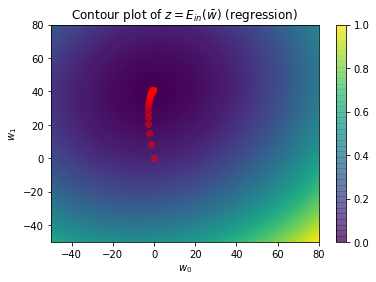In :
### This shows how the magnitude of the w your GD considered changed over time
### When could your program have stopped?

L = [mse_gradient(ww,X,y) for ww in path]
plt.title("Magnitude of gradient over time steps")
plt.xlabel("Time step")
plt.show()

Out: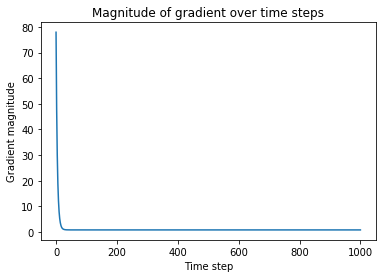In :
## This matrix version of MSE is from the bottom of pg 84 in the text
## It is 20 times faster than an implementation with python loops!

def MSE(w,X,y):
return 1/X.shape*(w.T.dot(X.T).dot(X).dot(w) - 2*w.T.dot(X.T).dot(y) + y.dot(y))

err_diffs = np.diff([MSE(ww,X,y) for ww in path])

plt.plot(np.arange(len(err_diffs)),err_diffs)
plt.title("Error differences over time")
plt.ylabel(r"$E_{in}(w_{t+1})-E_{in}(w_{t})$")
plt.xlabel("Time step")
plt.show()

Out: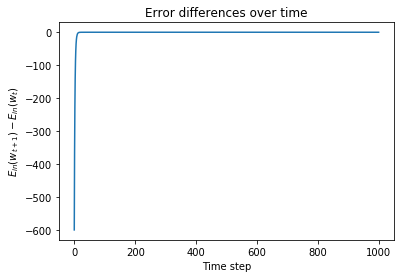### A real dataset¶

Let's see how your gradient descent performs on a real dataset, such as the Boston Housing data.

In :
import pandas as pd

Out:
0 1 2 3 4 5 6 7 8 9 10 11 12 13
0 0.00632 18.0 2.31 0 0.538 6.575 65.2 4.0900 1 296.0 15.3 396.90 4.98 24.0
1 0.02731 0.0 7.07 0 0.469 6.421 78.9 4.9671 2 242.0 17.8 396.90 9.14 21.6
2 0.02729 0.0 7.07 0 0.469 7.185 61.1 4.9671 2 242.0 17.8 392.83 4.03 34.7
3 0.03237 0.0 2.18 0 0.458 6.998 45.8 6.0622 3 222.0 18.7 394.63 2.94 33.4
4 0.06905 0.0 2.18 0 0.458 7.147 54.2 6.0622 3 222.0 18.7 396.90 5.33 36.2

### Uh Oh¶

We ran into some problems here.

Linear regression based on the normal equations is doing just fine, but gradient descent is struggling.

We had to put $\eta$ all the way down to 0.000001 just to get convergence.

But then after 100000 iterations, we still haven't converged to a good solution.

What's the problem?

Fix it! (Hint: What should you always do to the data before doing gradient descent? You can take the code for this operation from HW 2, or use the sklearn library version.)

When you fix it the output should look roughly like this:

w (normal equations )= [ 26.53112618 -10.30154035   4.11512828   2.44959865   2.11057155
-9.36246851  17.88281394   1.23610308 -13.15196355   7.6501691
-7.15185994  -9.86921614   3.64943825 -19.76860333]
R2 (normal equations) = 0.7165453029604523
w (gradient descent) = [ 19.9368617   -6.02022064   2.87944774   1.82739763   2.25551214
-6.37598968  22.22541246   1.55715796  -8.46547661   4.94478739
-4.63963461  -8.74471267   4.76136717 -18.60811081]
R2 (gradient descent)= 0.7123144123485858

You will need to put $\eta$ and max_iter back down to sane values to get this output.

In :
X_nb = df.values[:,:-1]
y = df.values[:,-1]
X = np.concatenate((np.ones(X_nb.shape).reshape(X_nb.shape,1),X_nb),axis=1)
X_train,X_test,y_train,y_test = train_test_split(X,y)
w = np.linalg.pinv(X_train).dot(y_train)
y_train_hat = X_train.dot(w)
y_test_hat = X_test.dot(w)

print("w (normal equations )= {}".format(w))
print("R2 (normal equations) = {}".format(R2(y_test,y_test_hat)))
w_init = np.random.randn(X.shape)

y_test_hat = X_test.dot(wgd)


w (normal equations )= [ 4.11421074e+01 -1.30942062e-01  5.22319928e-02 -1.04552172e-02
2.48575580e+00 -1.82902535e+01  3.19092537e+00  1.79515209e-02
-1.65793310e+00  2.89030013e-01 -9.57589737e-03 -9.61814615e-01
1.01109971e-02 -6.61234567e-01]
R2 (normal equations) = 0.7454904562072211
w (gradient descent) = [-1.32109713 -0.14188091  0.0335308  -0.01323346 -1.0983629  -1.48581662
1.89604917  0.13850109  1.01131218  0.14378951  0.00338265 -0.3105092
0.03266242 -0.73887929]

In :
err_diffs = np.diff([MSE(ww,X,y) for ww in path])

plt.plot(np.arange(len(err_diffs)),err_diffs)
plt.title("Error differences over time")
plt.ylabel(r"$E_{in}(w_{t+1})-E_{in}(w_{t})$")
plt.xlabel("Time step")
plt.show()

L = [mse_gradient(ww,X,y) for ww in path]
plt.title("Magnitude of gradient over time steps")
plt.xlabel("Time step")
plt.show()

Out: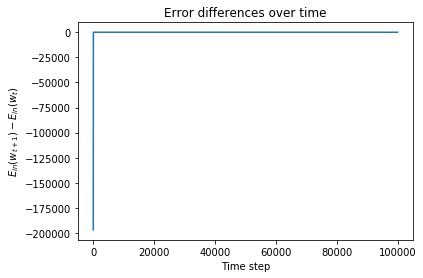Out: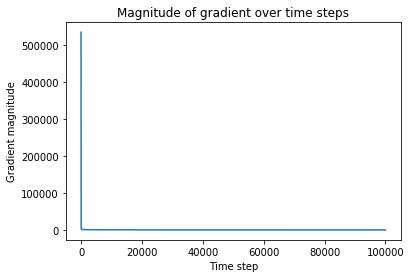In :
err_diffs[-10:]

Out:
array([-0.0001034 , -0.00010339, -0.00010339, -0.00010339, -0.00010339,
-0.00010338, -0.00010338, -0.00010338, -0.00010338, -0.00010338])
In :
gradients[-10:]

Out:
array([101.35818484, 101.35835124, 101.35851765, 101.35868406,
101.35885046, 101.35901687, 101.35918329, 101.3593497 ,
101.35951611, 101.35968253])

In this section you will implement stochastic gradient descent and apply it so some real data.

$\nabla {\bf e} = 2(\bar{w}^T\bar{x}_n-y_n)\bar{x}$

In :


"""parameters: x,w,yy  (instance, weight vector, truth value = +-1)
return:  new w
This is the gradient for MSE error.
The mathematical formula can be found above.
"""
### You complete me

"""parameters: w (initial weight vector)
X (data matrix)
y (target vector)

history = [] ## Every time you compute a new w, do history.append(w).
for j in range(num_epochs):
shuff = np.random.permutation(X.shape)
for i in range(X.shape):
#You complete me
history.append(w)
return w,np.array(history)


In :
### Be sure X_train and X_test are already scaled for this test.
### Actual R2 scores will vary but the normal eqns and sgd should give similar results.

w = np.linalg.pinv(X_train).dot(y_train)
yhat_test_ne = X_test.dot(w)
print("Solution from normal equations: \n",w)
print("R2_test(w) (normal equations) = {}".format(R2(yhat_test_ne,y_test)))

wsgd = np.random.randn(X.shape)
print("Solution from SGD")
yhat_test = X_test.dot(wsgd)
print("w = {},\n R2_test(w) (sgd) = {}".format(wsgd,R2(yhat_test,y_test)))

Solution from normal equations:
[ 28.80211923 -11.64954077   5.22319928  -0.28521832   2.4857558
-8.81590218  16.65343953   1.74309268 -18.23212448   6.64769029
-5.01777022  -9.04105739   4.00981923 -21.84719009]
R2_test(w) (normal equations) = 0.74643162250197
Solution from SGD
w = [ 28.75654953 -11.63512471   5.19798403  -0.22337634   2.43643442
-8.928694    16.38430564   1.7949666  -18.27312099   6.63783803
-5.10989417  -9.28696864   3.84921652 -21.80215601],
R2_test(w) (sgd) = 0.7421692098167241

In :
err_diffs = np.diff([MSE(ww,X,y) for ww in path])

plt.plot(np.arange(len(err_diffs)),err_diffs)
plt.title("Error differences over time")
plt.ylabel(r"$E_{in}(w_{t+1})-E_{in}(w_{t})$")
plt.xlabel("Time step")
plt.show()

L = [mse_gradient(ww,X,y) for ww in path]
plt.title("Magnitude of gradient over time steps")